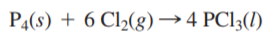×
Get Full Access to Chemistry: A Molecular Approach - 3 Edition - Chapter 4 - Problem 48e
Get Full Access to Chemistry: A Molecular Approach - 3 Edition - Chapter 4 - Problem 48e

×

# Elemental phosphorus reacts with chlorine gas according toISBN: 9780321809247 1

## Solution for problem 48E Chapter 4

Chemistry: A Molecular Approach | 3rd Edition

• Textbook Solutions
• 2901 Step-by-step solutions solved by professors and subject experts
• Get 24/7 help from StudySoup virtual teaching assistantsChemistry: A Molecular Approach | 3rd Edition

4 5 1 269 Reviews
31
0
Problem 48E

Elemental phosphorus reacts with chlorine gas according to the equation:A reaction mixture initially contains 45.69 g P4 and 131.3 g Cl2. Once the reaction has occurred as completely as possible, what mass (in g) of the excess reactant is left?

Step-by-Step Solution:
Step 1 of 3

Solution: Here, we are going to determine the oxidation numbers of sulfur in the given set of compounds. Step1: Oxidation number denotes the oxidation state of an element in a compound ascertained according to a set of rules. These rules are: 1. In elements, in the free or the uncombined state, each atom bears an oxidation number of zero. Evidently each atom in H2, O2, Cl2, O3, P4, S8, Na, Mg, Al has the oxidation number zero. 2. For ions composed of only one atom, the oxidation number is equal to the charge on the ion. In their compounds all alkali metals have oxidation number of +1, and all alkaline earth metals have an oxidation number of +2. Aluminium is regarded to have an oxidation number of +3 in all its compounds. 3. The oxidation number of oxygen in most compounds is –2. 4. The oxidation number of hydrogen is +1, except when it is bonded to metals in binary compounds (that is compounds containing two elements). 5. In all its compounds, fluorine has an oxidation number of –1. Other halogens (Cl, Br,and I) also have an oxidation number of –1, when they occur as halide ions in their compounds. 6. The algebraic sum of the oxidation number of all the atoms in a compound must be zero. In polyatomic ion, the algebraic sum of all the oxidation numbers of atoms of the ion must equal the charge on the ion. Step2: Based upon these rules, let us calculate the oxidation number of sulfur in the above given compounds: a) Barium Sulfate, BaSO 4 Since barium sulfate is a neutral molecule, so, sum of oxidation numbers of all its atoms will be zero. Thus, (+2) + x + 4(-2) = 0 [x being the oxidation number of sulfur] 2 + x - 8 = 0 x = 8 - 2 x = +6 Thus, the oxidation number of sulfur in barium sulfate is +6. b) Sulfurous acid, H 2 3 Since, sulfurous acid is a neutral molecule, so, sum of oxidation numbers of all its atoms will be zero. Thus, 2(+1) + x + 3(-2) = 0 [x being the oxidation number of sulfur] 2 + x - 6 = 0 x = 6 - 2 x = +4 Thus, the oxidation number of sulfur in sulfurous acid is +4. c) Strontium sulfide, SrS Since strontium sulfide is a neutral molecule, so, sum of oxidation numbers of all its atoms will be zero. Thus, (+2) + x = 0 [x being the oxidation number of sulfur] 2 + x = 0 x = - 2 Thus, the oxidation number of sulfur in strontium sulfide is -2. d) Hydrogen sulfide, H S 2 Since hydrogen sulfide is a neutral molecule, so, sum of oxidation numbers of all its atoms will be zero. Thus, 2(+1) + x = 0 [x being the oxidation number of sulfur] 2 + x = 0 x = - 2 Thus, the oxidation number of sulfur in hydrogen sulfide is -2. Step3: e) The atomic number of sulfur is 16. Hence, it is placed in the 16th column and 3rd row of the periodic table i.e., it is located in the region (D) of the given figure. Step4: f) Nonmetals are the elements which can adopt both positive and negative oxidation numbers. Hence, region (D) contains the elements which can adopt both positive and negative oxidation numbers. For example, Sulfur is an element of region (D) and is a nonmetal. The oxidation number of sulfur in sulfurous acid is +4 whereas in hydrogen sulfide is -2. ------------

Step 2 of 3

Step 3 of 3

##### ISBN: 9780321809247

Unlock Textbook Solution